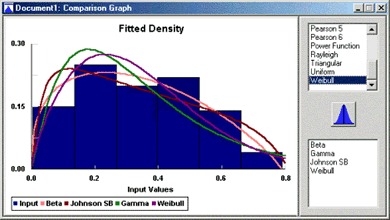# Stat::Fit for SIMUL8 - \$245

Stat::Fit for SIMUL8 is SIMUL8 Basic Plug-in that fits your data to the most useful analytical distribution. Stat::Fit for SIMUL8 is included with SIMUL8 Professional.

Buy | Money Back Guarantee

Stat::Fit will take your raw data from spreadsheets, text files, or manual input and convert that data into the appropriate distribution for instant input into SIMUL8. Stat::Fit provides relative comparisons between distribution types, and an absolute measure of each distribution's acceptability.Features include descriptive statistics, parameter estimates, goodness of fit tests, graphical analysis, random variate generation, export to simulation products, and more.

Distribution Fitting

• Automatic or Manual fitting.
• Includes 15 distributions:
Beta, Binomial, Erlang, Exponential, Gamma, Gauss, Log Normal, Negative Binomial, Normal, Pearson V, Pearson VI, Poisson, Triangular, Uniform, Weibull.

Descriptive Statistics

• Mean
• Median
• Mode
• Standard Deviation
• Variance
• Coefficient of Variation
• Skewness
• Kurtosis

Parameter Estimates

• Maximum Likelihood
• Moments

Goodness of Fit Tests

• Chi-squared
• Kolmogorov-Smirnov
• Anderson-Darling

Graphical Analysis

• Density graphs
• Distribution Graphs
• Difference graphs
• Box Plots
• Q-Q plot
• P-P plot
• Scatter plot
• Autocorrelation graphs

Additional Features

• Built-in random variate generator
• Data manipulation options
• Distribution Viewer